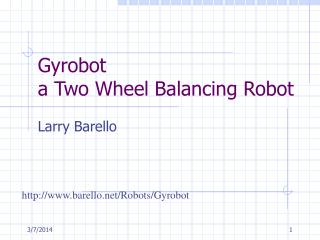# Gyrobot a Two Wheel Balancing Robot - PowerPoint PPT PresentationDownload PresentationGyrobot a Two Wheel Balancing Robot

Gyrobot a Two Wheel Balancing Robot
Download Presentation## Gyrobot a Two Wheel Balancing Robot

- - - - - - - - - - - - - - - - - - - - - - - - - - - E N D - - - - - - - - - - - - - - - - - - - - - - - - - - -
##### Presentation Transcript

1. Gyrobota Two Wheel Balancing Robot Larry Barello http://www.barello.net/Robots/Gyrobot

2. How Balancing Works • Sense tilt and drive wheels to make robot erect. • Easier to balance high GC object. • The Devil is in the details. Balanced Tilted

3. How Balancing Works • Restoring Torque =  * K •  = Angular rate (deg/sec) • K = some factor based upon robot mass & moment arm.

4. M M g Motion M T = M * g * sin(0) T = M * g * sin(90) How Balancing Works • Balance torque = M * g * sin(angle) • M is moment arm (center of mass & distance from pivot). • g = acceleration of gravity • Angle is deviation from balance point

5. Controlling Drive Torque • As velocity increases, DC motors have less torque (back-EMF) • Need to correct this so balance equation can work. • Positive velocity feedback • Drive = drive + Kv * velocity • Kv = factor based upon motor specification.

6. How to move • The robot tilts in the direction of movement. • To move robot, need to adjust “balance angle” • By adding an error to the angle, the robot will drive a little backwards, then forwards trying to maintain the “balance angle” • Error can be simply a negative drive value.

7. Tilt Sensor • Gyroscope measures Rate Of Turn. • Integrate for angle • Gyroscopes drift • Drift changes withtemperature

8. Tilt Sensor • Accelerometers measure gravity. • Affected by movement. • Very accurate.

9. Fusing Gyro and Tilt sensors • Weighted Average Filter. • Use fraction of error between tilt and gyro • Add to integrated Gyro output. • Results are fast & drift free • The weighted average IIR filter technique can be used to smooth out any noisy signal.

10. Fusing Gyro and Tilt sensors • Weighted Average Filter. • Tiltgyro = Sum(Gyro – Offset) • Tiltaccel = sin-1(x/g) • Tiltgyro = Tiltgyro + (Tiltaccel – Tiltgyro) * K + + Gyro  Tilt - - Offset + Accelerometer K K = weighting factor (1%)

11. Alternate to Tilt Sensor • F = g * sin(theta) • F = A * m • A * M = g * sin(theta) • Theta = sin-1(A * m/g) • m = mass • A = Acceleration M/S2 • g = Gravity • F = force Presumably we can use the acceleration of the robot to infer the angle of tilt and correct for gyro drift. (Note: Moment arm not included, formulas must be wrong)

12. Motion Sensor • Encoders measure position and velocity of wheels. • Dead reckoning can be derived from encoders and the robots position determined in X, Y and Theta.

13. Current Status • Motion control not implemented. • Navigation disabled. • Remote control (Radio link) not implemented. • Using acceleration to correct tilt not tried. In short, it doesn’t do anything interesting yet.